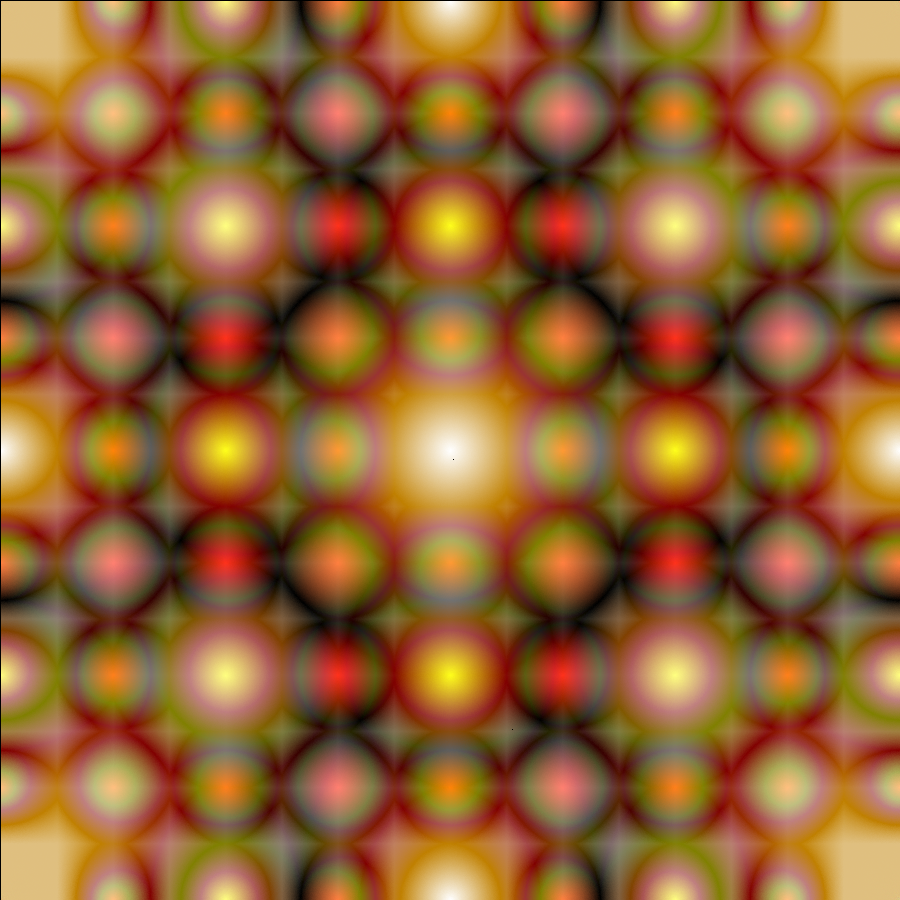## ↩ Tonči Juričev-Grgin mandalas

The Tonči Juričev-Grgin mandala generation algorithm can be used to, drumroll please, generate mandalas like this one:Click for big version.

It uses a very simple process (see below), which can be used in any [​[​beta in NN ∖ {0}​]​]-dimensional space, so, as an example, the same-sized mandala, but in one more dimension (also with classical music atop it because it'd be unbearably bloody boring otherwise):

"How long does rendering a thing such as this take?", one might ask. Two answers, the short one - too sodding long, the not-quite-as-short one is that due to the process' inherent randomness one need run the algorithm body many times, an acceptable amount I found was:

[​[​log(n_max) = |~log(prod_i size_i)~| + 1​]​],

(which still doesn't perfectly fill more complex corner setups), so for [​[​size = [900, 900, 900] => n_max = 10^10​]​], which takes about [​[​7 1/2 h​]​] on my PC if I try to not cook it (and I generally do).

A different question to be asked is "How does one laugh at the shitty code play around with the code that generates these myself?". Well, thanks to the wonders of the [​[​fr "Internet"​]​], you can! In most-to-least bad order:

Here's an early version in C++, featuring layers upon layers of terrible code from late-2015/early-2016. You probably don't want to try to build it, headaches afore.

Here's the very first version, in JavaScript, used to demostrate as PoC to Tonči himself. You probably want to fuck around, if at all, in that version (download as ZIP).

Here's a pretty-much release version in Rust, used to generate all here-featured examples. You probably want to use this if you want to generate your own mandalas or make things based on the algorithm. Will work and build pretty much anywhere.

"I don't care, I just wanna look at more cool shit!" — I got you covered on this front, too:

Here's just a lot of mandalas in various sizes, colours, and shapes. Make sure to be on Wi-Fi, because they get really, really big in a couple places.

Here's a playlist with some 3-dimensional mandalas and classical music atop.

I'm out of things to put here, because I'm not a cool person en general. (What else did you expect, I do maths for fun)

"I like/don't like this thing and/or would like to tell/contact you about it/something else.", then reach me over at anything listed here. I don't have a life so I'll probably respond uncannily quickly.

"But where's the algorithm?" – Here's the raw version:

[​[​sf "Mandala Generation - Ton" hat(sf "c") sf "i Juri" hat(sf "c") sf "ev-Grgin"​]​]

[​[​alpha in NN ∖ {0} - sf "colour space dimensionality (e.g. 3 for RGB, 4 for CMYK, 1 for LUMA)"​]​]

[​[​c nn (: 3, +oo ) - sf "amount of corners in c-gonal mandala base"​]​]

[​[​C - sf "set of point" - sf "corner points of c-gon mandala base"​]​]

[​[​bar C = c​]​]

[​[​n in NN nn (: alpha + 2; +oo ) - sf "current point number"​]​]

[​[​d = ra\nd((:0, c) nn NN) - sf "current chosen corner"​]​]

[​[​P_n = (C_d + P_(n-1)) / 2 => a = |C_d P_(n-1)| and b = a/2 = |C_d P_n| = |P_(n-1) P_n|​]​]

[​[​e_m = |P_n P_(n-m)|​]​]

[​[​E = so\rt(map(f(x) = e_x, NN nn (: 1; alpha + 1 :)))​]​]

[​[​colour P_n = map(f(x) = E_x / E_alpha; E ∖ {E_alpha})​]​]

[​[​or​]​]

[​[​f = max E​]​]

[​[​colour P_n = map(f(x) = x / f; E ∖ {f}) => bar(E ∖ {f}) = alpha​]​]

(I'm not a real mathematician, so it's most likely not done properly.)

And here's a scanned hand-drawn version with examples (warning: very big, expand at own risk).

On some of the more arbitrary colourspaces.

Since the output image format (PNG) and the video format (H.264) only support RGB (nota bene: I am omitting a scheissload of detail and specificites I either do not know or that are not relevant because colours are hard) a conversion is required when going from e.g. CMYK or YUV thereto. More often than not (which is to say, in all spaces but LUMA), that conversion is lossy, which means that "in colourspace" is a lie, whereas the non-lie version is closer to "in an RGB approximation to colourspace". However, most conversions were already set in stone well before I was born and are therefore widely accepted as accurate enough. Some, however, like ROYGBP or, ahem, RPPDIBLCTGLLYAODBGB, have been made up by yours truly upon the following basis:

[​[​S - sf "set of 3-dimensional vectors" - sf "RGB samples of each colour in a space"​]​]

[​[​bar S = alpha​]​]

[​[​colour P_n - sf "as above"​]​]

[​[​AA c in {r,g,b} : C_(out_c) = (sum_(theta=1)^alpha colour P_(ntheta) S_(theta_c))/(sum_(theta=1)^alpha S_(theta_c))​]​]

A full list of conversions and their implementations can be found at the mandalas-rs API documentation.